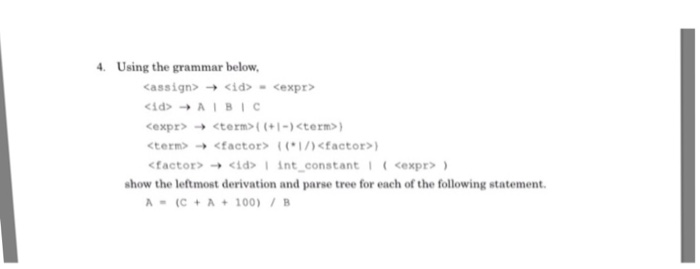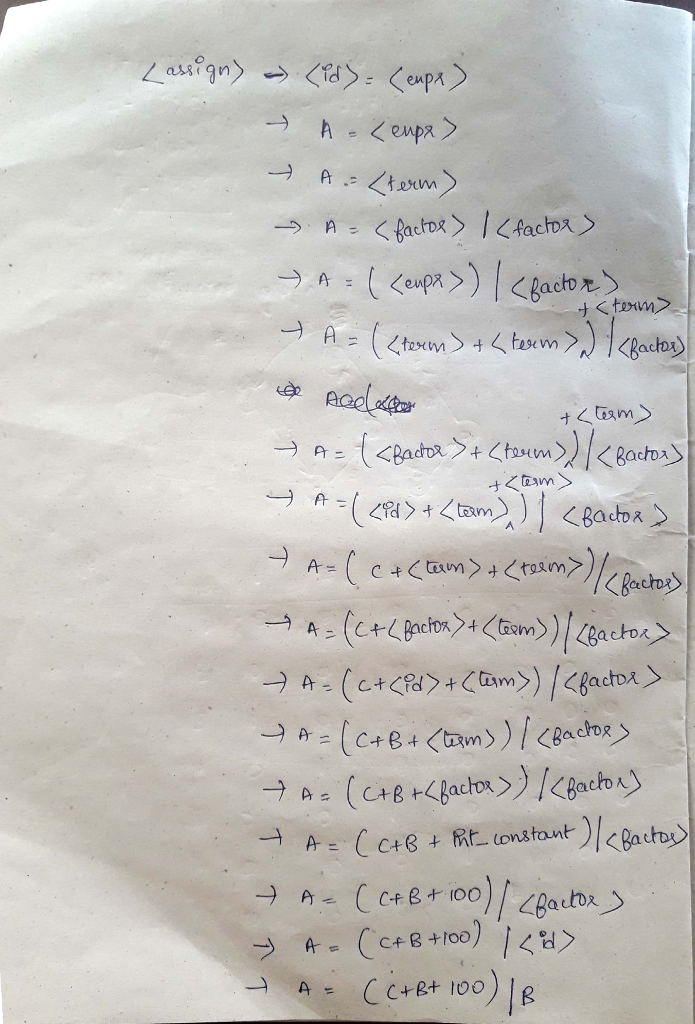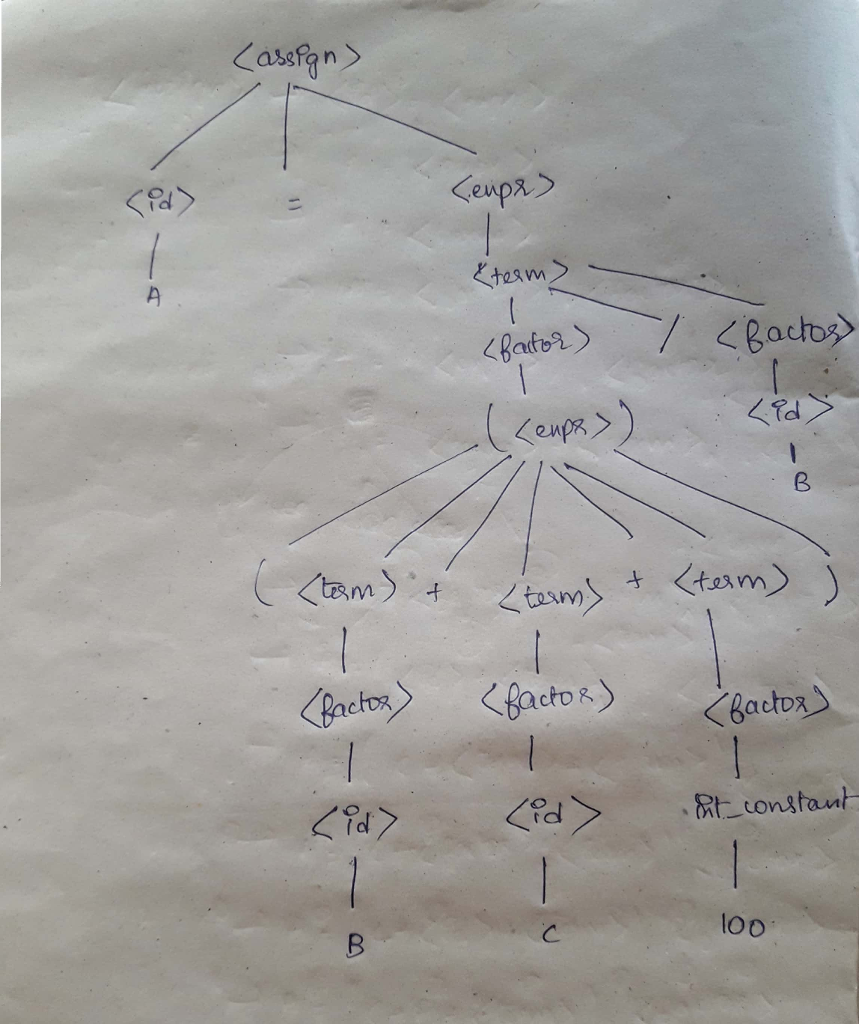# Homework Solution: Using the grammar below. rightarrow rightarrow A | B | C rightarrow {(+|-) ) rightarro…Using the grammar below. rightarrow rightarrow A | B | C rightarrow {(+|-) ) rightarrow (( /) ) rightarrow int_constant ( ) show the leftmost derivation and parse tree for each of the following statement. A = (C + A + 100)/B